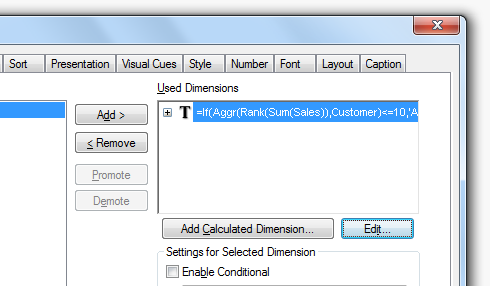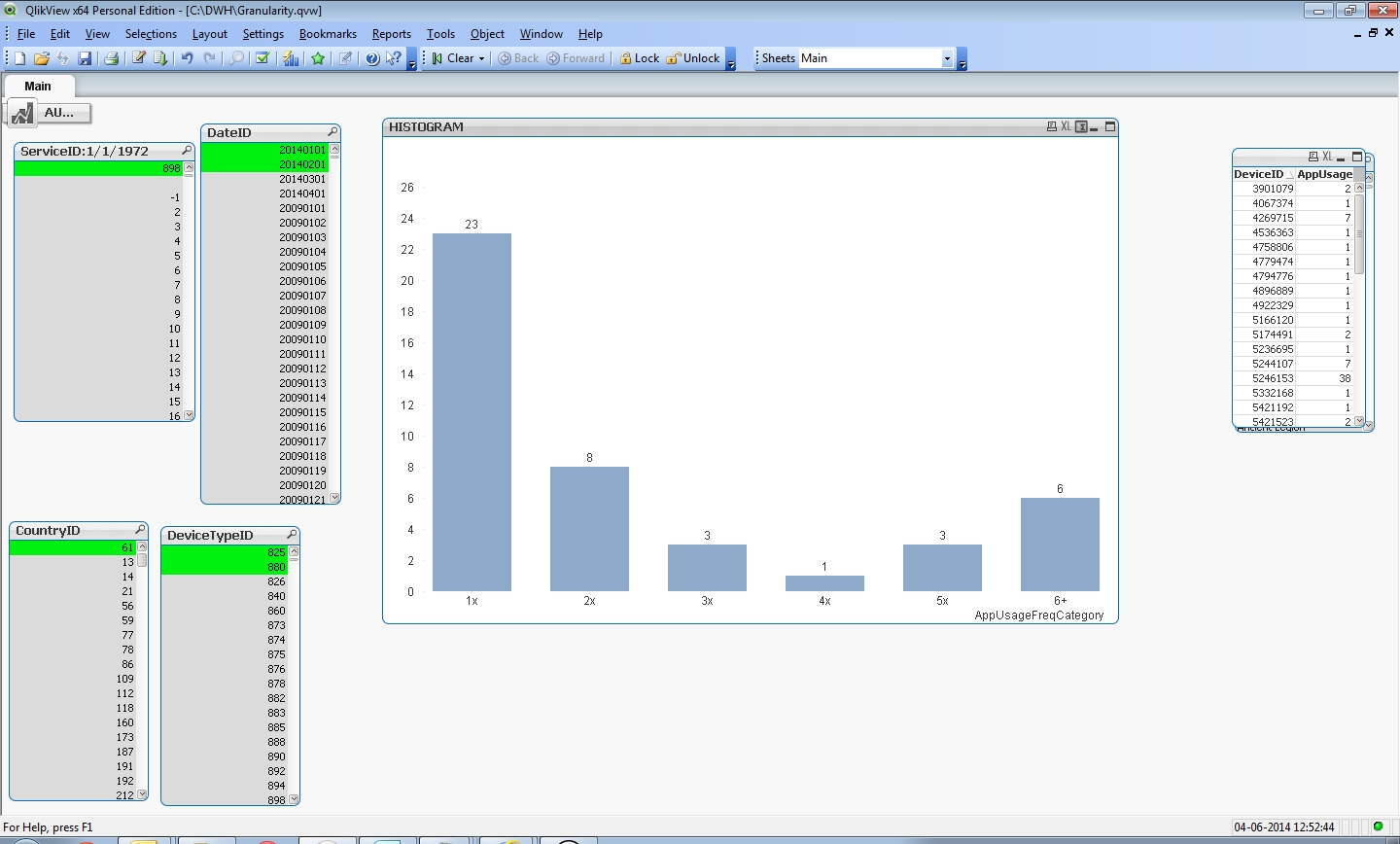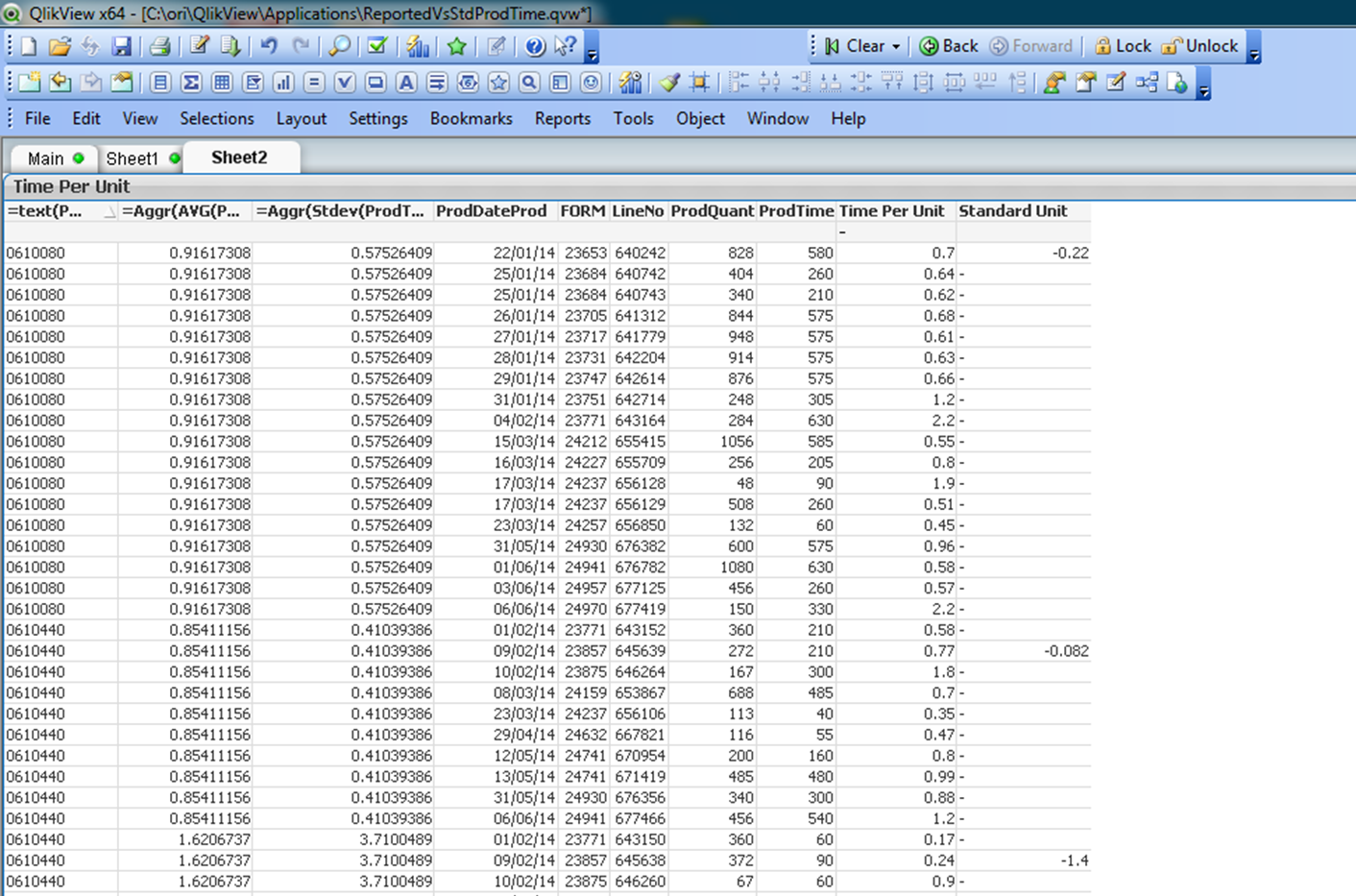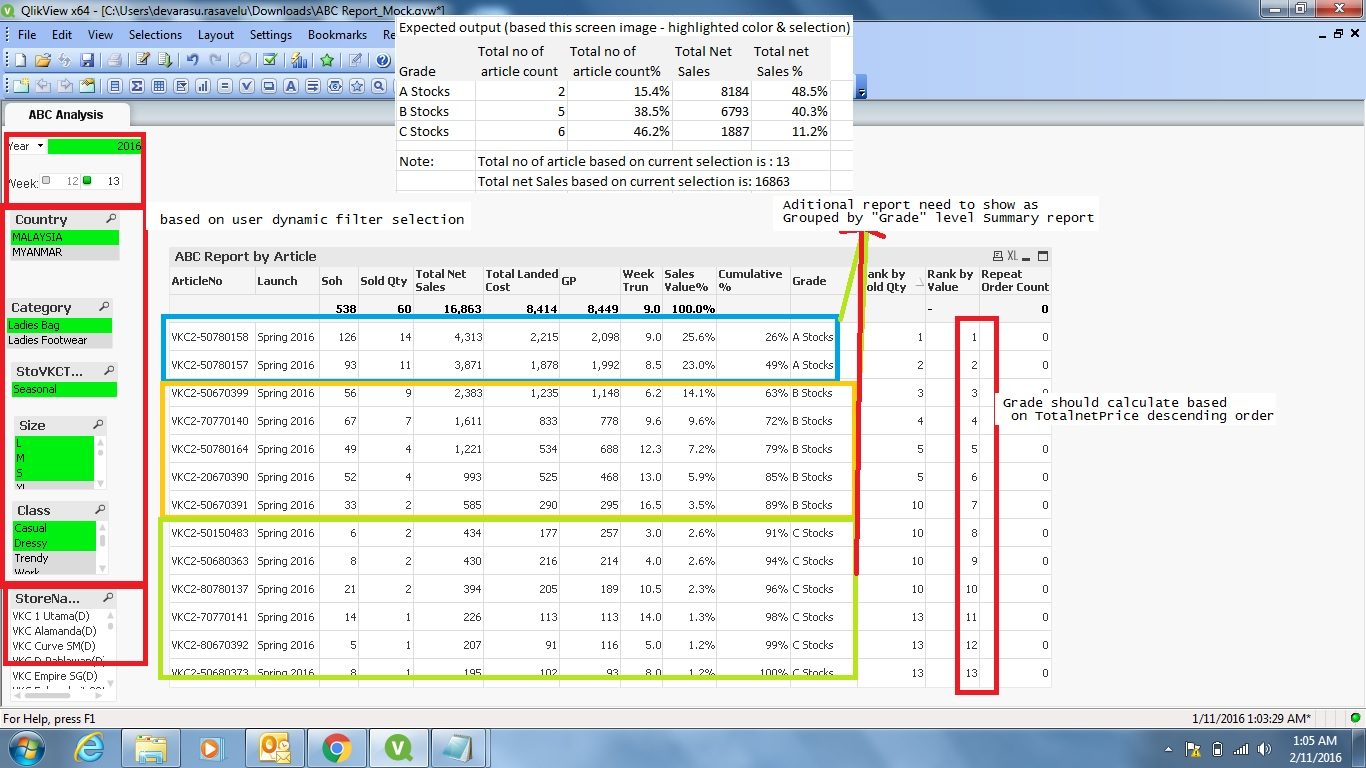# Qlik Design Blog

All about product and Qlik solutions: scripting, data modeling, visual design, extensions, best practices, etc.Employee

# Calculated Dimensions

To make a chart in QlikView – in any Business Intelligence tool, for that matter – you need to have one or several dimensions; entities with discrete values that you use as grouping symbols. But where should you define these dimensions: In the script or in the object where the dimension is used?

In most cases, you will use an existing field as dimension, i.e. an attribute that exists in the source data. In such a case, the answer to the above question is easy: Just make sure to load the field in the script, and you're done.

But in some cases you want to use derived attributes: Attributes that do not exist in the source data but one way or another can be calculated from existing fields.

One example is the fields of the Master Calendar: Year, Month, etc. These can all be derived from a date found in the source data:

Month(Date) as Month

Year(Date) as Year

A more advanced example is if you want to classify or rank a field. The following expression returns ‘A’ for the 10 best customers and a ‘B’ for the rest:

If(Aggr(Rank(Sum(Sales)),Customer)<=10,'A','B')

For such fields the above question is very relevant: Should they be calculated in the script and saved as fields, or should they be calculated on the fly in a sheet object?There are pro:s and con:s with both approaches: A field calculated in the script is calculated once and for all, so it does not need to be re-calculated every time the user clicks. Hence, response times will be slightly shorter if the field is calculated in the script.

On the other hand, in some cases you want the field to be re-calculated every time the user clicks. A good example is the classification using Rank() above. Most likely you want this field to depend on the selection made: If you have selected a product, you want to see the classification of the customers given this selection. Such a number is in its nature dynamic and should be calculated every time the user clicks.

The key is whether the calculated field should be static or dynamic. The field Month is static: A specific date always belongs to the same month, irrespective of user selection. As opposed to a classification or a rank where the calculation usually should be dynamic, since the result potentially could change every time the user clicks.

Bottom line is that dynamic fields must be calculated in the chart or the list box. But for static fields it is better if they are calculated in the script, since precious CPU-time otherwise will be unnecessarily spent every time the user clicks.

HIC

Note: The Rank() function cannot be used in the script, so if you want to calculate a static rank in the script, you need to do it in a different way, using e.g. a combination of Order By and RecNo().

Further reading related to this topic:

Buckets

25 CommentsPartner

hi HIC,

nice

3,441 ViewsNot applicable

Thank you for sharing this valuable insight.

3,441 ViewsMVP

Good!!

3,441 ViewsLuminary

Thanks HIC. No doubt Calculations Dimensions have their place for usage. What are the implications on caching when we use the Calculated Dimension? I guess it would have negative impact on the cache..?

Thanks,

DV

3,441 ViewsEmployee

Yes, they have an impact on the cache. But I wouldn't say that it is negative... The result of a calculated dimension will be stored in the cache and re-used properly. Which means that if you have the same data set, the same selection and the same expression, a look-up will be made in the cache to save CPU-time.

HIC

3,441 ViewsNot applicable

Hi, thanks HIC! Its Good!.

3,441 ViewsNot applicable

Hi Nice Thanks.

3,441 ViewsNot applicable

Good to know this, i always heard that calculated dimensions wont cache hence it impacts performance. So can we safely assume calculated dimensions cache equivalent to regular dimensions?

3,441 ViewsEmployee

Calculated dimensions are definitively cached, just as any expression..

It is true that calculated dimensions sometimes are heavy to calculate, and thus impact performance. But if so - the more reason to cache them!

HIC

3,441 ViewsNot applicable

Thanks Henric, good post.

I wanted to achieve this, but as a Qlikview beginner, I wasn't sure how to do it.

Specifically I wanted to show a List View of States (in US), but only show the States with the top 5 sales.

1. My first instinct was, "Dimension limits", but these only apply in Chart Tables, not in List Views. Correct that Dimensnion limits could only help in Chart Tables?
2. My second instinct was, "Set analysis", because my problem suggets a solution which can "filter"; Set analysis allows me to filter with Modifier expression. I was thinking something like AGGR(ONLY( {1 <Sum(SALES) = { '> \$FifthHighestSales' } > } ) ). But I don't think Set analysis is appropriate because it doesn't work on aggregations. i.e. above gives "Error: Error in set modifier expression" Correct that set analysis is not the right tool for my problem? NOTE: Henric pointed out correct syntax below; the problem is that I used SUM(Sales) as the identifier; however, the SUM(SALES) Expression should be inside the modifier (i.e. between the brackets). Set can be used to solve the problem.
3. So I finally found your post here, and this post here. You made it clear how to solve the problem. I have only one curiosity, the IF condition: AGGR(RANK(SUM(SALES))) >=10 . I thought AGGR produces a set (i.e. multiple ) of values, whereas 10 is only a scalar (i.e. single value) . How is this statement evaluated? Is AGGR indeed producing a set? Or maybe AGGR is producing only a single value since the entire thing is evaluated as a Dimension, one-dimension-value-at-a-time? I hope my question is clear...
3,441 ViewsEmployee
1. You are right that Dimension Limits only exists in charts, so this option is not possible.
2. You can use Set Analysis. See below.
3. Aggr() does indeed produce several values. You need the second parameter, the grouping symbol, to define how many values it should produce.

You need a calculated dimension, but this you can have in a list box also. Just choose <Expression> from the drop down list. The following expressions are both possible:

=Aggr(If(Rank(Sum(Sales))<=5,Only(State)), State )

=Aggr(Only({1<State={"=Rank(Sum(Sales))<=5"}>} State), State )

HIC

3,441 ViewsNot applicableHello Henric,

Your explanation on Calculated Dimension helps a lot. I now understand that I need something like for the problem I'm facing.

I want to have a 'histogram' that works for selecting one single month, but also in case I select multiple (e.g. 2 months).

In this histogram on the X-axis I want to show the 'AppUsageFrequencyCategories': 1x, 2x, 3x, 4x, 5x, 6+x for nr of times a certain App has been used. This AppUsageFrequencyCategory is dependent on AppUsage attribute which contains how many time the App is used in a specific month for a specific country etc.

In the expression I calculate the number of devices (COUNT DISTINCT DeviceID) for which in a certain month the selected App (=ServiceID) has been 1x, 2x, 3x etc.

I first had the 'AppUsageFrequencyCategory' as a calculate attribute, but I do understand now I need a Calculated Dimension for it.

Simply example of the problem why my currently defined Calculated Dimension does not seem to work:

For Month 20140101:

DeviceID, AppUsage

7602767 2

7603047 2

8538589 9

For Month 20140201:

DeviceID, AppUsage

7602767 1

8102376 2

8538589 13

I get:

For Month 20140101 and 20140201 (both selected):

DeviceID, AppUsage

7602767 2

7603047 2

8102376 2

8538589 9

8538589 13

I need:

For Month 20140101 and 20140201 (both selected):

DeviceID, AppUsage

7602767 3 (1+2)

7603047 2

8102376 2

8538589 22 (9+13)

And because "I get" (see above) is not what "I need", the histogram will count the number of Unique Devices wrongly as it does a count distinct devices within the AppUsageFrequency Categories. I see that I have some double counting if in the first month the App Usage for a unique Device is in a different 'category' then in the second month etc.

My Calculated Dimension is the following:

=IF(AppUsage=1,'1x',IF(AppUsage=2,'2x',IF(AppUsage=3,'3x',IF(AppUsage=4,'4x',IF(AppUsage=5,'5x','6+')))))

The problem is that I need to do the SUM or AGGR over the selection (e.g. the two months) so that a DeviceID that uses that App e.g. 1x in Month 1 and 1x in Month 2 is calculated as '2x' and not as '1x'.

Before I had the following line as a calculated attribute in my table (which work if I select only one month):

IF(AppUsage=1,'1x',IF(AppUsage=2,'2x',IF(AppUsage=3,'3x',IF(AppUsage=4,'4x',IF(AppUsage=5,'5x','6+')))))  AS AppUsageFreqCategory

Any suggestion?

If needed I can provide the source code, or a picture of the current QV model I have.

3,441 ViewsEmployee

I think you need something along:

Aggr(Count(distinct DeviceID),Month,ServiceID)

and if you want to limit it to the numbers between 0 and 6, you can wrap the Count() in a RangeMin() function:

Aggr(RangeMin(Count(distinct DeviceID),6),Month,ServiceID)

HIC

3,441 ViewsNot applicable

Hi Henric, really nice, I have already use it to do the rank Top 40! It looks good. Thank you!

3,441 ViewsNot applicable

Hi Henric, I'm confronting some problems in this subject, hope You can help :

I want to calculate a dynamic statistical standard unit.

The production line is producing different parts, each part has its own average production time and standard deviation. The reported production time for each part is saved in the SQL server.

In order to calculate the histogram for the whole production line (not for a single part) I need to calculate the AVG and STDV for each part, then, for each reported part calculate  (Xi-AVG)/STDEV.

In order to keep the report dynamic I need to do the calculations in a sheet object ,not in the script.

The problem is, when I use AGGR function to calculate AVG and STDEV for a set of reported production time for a specific part  and subtract the specific reported time,

QlikView calculates only the first row.

This is the formula I use (in the expression or in the dimension)  :

=(ProdTime/ProdQuant - Aggr(Avg(ProdTime/ProdQuant),PART))/Aggr(Stdv(ProdTime/ProdQuant),PART)Any suggestion?

3,441 ViewsEmployee

Yes, the Aggr() function can be a little grumpy...You need to have the same grain in the entire formula. Let's say that you make multiple measurements per part, and you have multiple parts in each Plant/Month/Division. Each measurement has exactly one ProdTime and one ProdQuant.

Then I would do a:

=Aggr(

Only(ProdTime/ProdQuant)

- Avg( total <Part> ProdTime/ProdQuant) / Stdev( total <Part> ProdTime/ProdQuant),

Measurement, Part)

as my basic calculation. Note that the Avg() and the Stdev() are calculated for a larger set than the Only() function: It uses all measurements for that Part.

If you round this number to nearest integer (or whatever is appropriate) you can make a histogram using the rounded number as dimension.

HIC

PS I have in fact already written a blog post about box plots that uses such a calculation. It will be published later during the summer.

3,441 ViewsNot applicable

Thanks a lot  for perfect and fast solution,

Building a histogram with Class function was not the problem. only the AGGR calculation.

Using this function  Avg(TOTAL <PART,ACT> ProdTime/ProdQuant) instead of AGGR is the solution.

This is the whole function :

=(ProdTime/ProdQuant- Avg(TOTAL <PART,ACT> ProdTime/ProdQuant))/Stdev(TOTAL <PART,ACT> ProdTime/ProdQuant)

Can you explain this part of the function : TOTAL <PART,ACT> or send a link to a relevant blog?

Ori Donner.

3,441 ViewsEmployee

I should probably write a blog post on that....

Anyway, if you have a chart or an aggr() fucntion, you can use the total qualifier to disregard the dimension(s).

if you have a pivot table with month and region, then

Sum(total Sales) is the sum over the entire chart.

Sum(total <month>) is the sum of the row

Sum(total <region>) is the sum of the column

See also

The Aggregation Scope

When should the Aggr() function NOT be used?

HIC

3,441 ViewsNot applicable

Thanks, waiting for your blog.

3,441 ViewsNot applicable

By using a calculated dimension it takes more time when compared to normal dimension is there any function or any application that shows the time taken for each chart each object

3,441 ViewsEmployee

You can create a memory statistics application. In it you have a field CalcTime that will give you a fair estimate. Note however, that if the object calculation is cached, the CalcTime will be zero.

HIC

3,441 Views
Contributor

hi

in calculated dimension can we use conditions

give me exp....

3,441 ViewsEmployee

What I use in the blog post, is in fact a calculated dimension with a condition:

If(Aggr(Rank(Sum(Sales)),Customer)<=10,'A','B')

You can also have conditions in other ways. See e.g. my post on Buckets where I use a Pick() function for my condition.

HIC

3,441 Views
Contributor

Hi.

Thank you for this post. I just want to add that there is one problem at calculated dimensions which you need to have in mind using them: be careful and avoid to use directly two or more dimensions on row-detail-level (i.e. end-start as difference). If you click in the chart you would select in all used dimensions.

3,441 Views
Honored Contributor II

Hi,

Got requirement like below

need to calculate the grade based on calculated measure and user selected dynamic filter value.

Let's assume we have list box filter as country,Category,Week,size etc, Based on the dimension we need to calculate the Grade (Calculated dimension). would it be possible to derive below summary report by Gradeproblem statement:

The problem with calculated dimension is that unable to sort the RangeSum in the descending order and it's creating incorrect Grades.

For more details, i have shared my qlikview file in below thread,

Re: ABC Analysis in Qlikview

Thanks Deva

3,441 Views